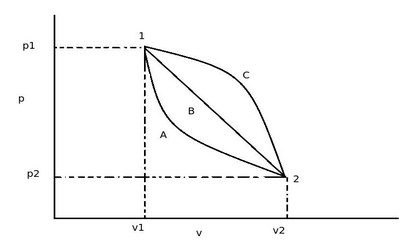# Work involved in a thermodynamic process is.

## Work involved in a thermodynamic process is

a. a path function
b. a point function
c. a transfer function
d. none of the above

Correct Answer : a. a path function

Explanation :As shown in above diagram, the thermodynamic system can be taken from state 1 to state 2 along many quasi-static paths like path A, B or C. The area under the curve indicates the amount of work done for each process. But those areas under each curve is different. Therefore the work involved does not depend upon the final state B but it depends upon the path by which the system is taken to the final state. Thus the work involved in thermodynamic process is a path function.